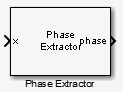# Phase Extractor

Extract the unwrapped phase of a complex input

• Library:
• DSP System Toolbox / Signal Operations

•## Description

The Phase Extractor block extracts the unwrapped phase of a complex input.

## Ports

### Input

expand all

Specify the input signal as a vector or a matrix. When the input is a matrix, the block treats each column of the signal as an independent channel. The first dimension is the length of the channel. The second dimension is the number of channels. The block treats one dimensional inputs as one channel.

Data Types: `single` | `double`
Complex Number Support: Yes

### Output

expand all

The block returns the unwrapped phase of the signal.

The block preserves the input size and dimension, and the output port rate equals the input port rate.

Data Types: `single` | `double`

## Parameters

expand all

When you clear this check box, the block ignores boundaries between the input frames. When you select this check box, the block treats each frame of input data independently, and resets the initial phase value for each new input frame.

Specify the type of simulation to run as one of the following:

• `Code generation` –– Simulate model using generated C code. The first time you run a simulation, Simulink® generates C code for the block. The C code is reused for subsequent simulations, as long as the model does not change. This option requires additional startup time but provides faster simulation speed than `Interpreted execution`.

• `Interpreted execution` –– Simulate model using the MATLAB®  interpreter. This option shortens startup time but has slower simulation speed than `Code generation`.

## Block Characteristics

 Data Types `double` | `single` Direct Feedthrough `no` Multidimensional Signals `no` Variable-Size Signals `yes` Zero-Crossing Detection `no`

## Algorithms

Consider an input frame of length N:

`$\left(\begin{array}{l}{x}_{1}\\ {x}_{2}\\ ⋮\\ {x}_{N}\end{array}\right)$`

The `step` method acts on this frame and produces this output:

`$\left(\begin{array}{l}{\Phi }_{1}\\ {\Phi }_{2}\\ ⋮\\ {\Phi }_{N}\end{array}\right)$`

where:

`${\Phi }_{i}={\Phi }_{i-1}+\text{angle}\left({x}_{i-1}^{*}{x}_{i}\right)$`

Here, i runs from 1 to N. The `angle` function returns the phase angle in radians.

If the input signal consists of multiple frames:

• If you set `TreatFramesIndependently` to `true`, the `step` method treats each frame independently. Therefore, in each frame, the `step` method calculates the phase using the preceding formula where:

• ${\Phi }_{0}$ is 0.

• ${x}_{0}$ is 1.

• If you set `TreatFramesIndependently` to `false`, the `step` method ignores boundaries between frames. Therefore, in each frame, the `step` method calculates the phase using the preceding formula where:

• ${\Phi }_{0}$ is the last unwrapped phase from the previous frame.

• ${x}_{0}$ is the last sample from the previous frame.

## Version History

Introduced in R2014b